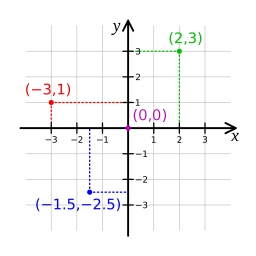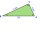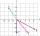# A Cartesian framework

1. In a Cartesian framework, the functions f and g we know that:
the function (f) is defined by f (x) = 2x ^ 2, the function (g) is defined by g (x) = x + 3, the point (O) is the origin of the reference, point (C) is the point of intersection of the graph of the function (g) with the ordinate axis, points A and B are the points of intersection of the graphs of the functions (f) and (g)
1.1 write the coordinates of points (A) and (B)
1.2. indicate the solutions of the equation f (x) = g (x)
1.3. determine the area of the triangle [OAC]
2.1. In the figure, part of the graph of a quadratic function (f) of the type: f (x) = ax ^ 2, a related function (g) and the trapezium [OBAC] are represented in a Cartesian framework. :
point (O) is the origin of the reference, point (B) is the point of intersection of the graph of the function (g) with the ordinate axis equal to 6, point A is the point of the intersection of the graphs of the functions (f) and (g)
point (C) belongs to the abscissa axis and has abscissa equal to 4, the trapezium area [OBAC] is equal to 18
2.1 Determine the coordinates of the point (A)
2.2. Determine the algebraic expressions of the functions (f) (g)

Ax =  1.5
Ay =  4.5
Bx =  -1
By =  2
Cx =  0
Cy =  3
S =  2.25

### Step-by-step explanation:

Our quadratic equation calculator calculates it.

$Ay={A}_{y}={A}_{x}+3=1.5+3=4.5$
$Bx={B}_{x}={x}_{2}=\left(-1\right)=-1$
$By={B}_{y}={B}_{x}+3=\left(-1\right)+3=2$
$Cy={C}_{y}={C}_{x}+3=0+3=3$Did you find an error or inaccuracy? Feel free to write us. Thank you!Tips to related online calculators
Line slope calculator is helpful for basic calculations in analytic geometry. The coordinates of two points in the plane calculate slope, normal and parametric line equation(s), slope, directional angle, direction vector, the length of the segment, intersections of the coordinate axes, etc.
Looking for help with calculating roots of a quadratic equation?
Do you have a linear equation or system of equations and looking for its solution? Or do you have a quadratic equation?
Pythagorean theorem is the base for the right triangle calculator.
Do you want to convert length units?

#### You need to know the following knowledge to solve this word math problem:

We encourage you to watch this tutorial video on this math problem:

## Related math problems and questions:

• Three pointsThree points K (-3; 2), L (-1; 4), M (3, -4) are given. Find out: (a) whether the triangle KLM is right b) calculate the length of the line to the k side c) write the coordinates of the vector LM d) write the directional form of the KM side e) write the d
• On a lineOn a line p : 3 x - 4 y - 3 = 0, determine the point C equidistant from points A[4, 4] and B[7, 1].
• Vertex pointsGiven the following points of a triangle: P(-12,6), Q(4,0), R(-8,-6). Graph the triangle. Find the triangle area.
• Unit vector 2DDetermine coordinates of unit vector to vector AB if A[-6; 8], B[-18; 10].
• Vertices of a right triangleShow that the points D(2,1), E(4,0), F(5,7) are vertices of a right triangle.
• Medians and sidesTriangle ABC in the plane Oxy; are the coordinates of the points: A = 2.7 B = -4.3 C-6-1 Try calculate lengths of all medians and all sides.
• Points on circleIn the Cartesian coordinate system with the origin O is a sketched circle k /O; r=2 cm/. Write all the points that lie on a circle k and whose coordinates are integers. Write all the points that lie on the circle I / O; r=5 cm / and whose coordinates are
• Equation of circle 2Find the equation of a circle that touches the axis of y at a distance of 4 from the origin and cuts off an intercept of length 6 on the axis x.
• General line equationsIn all examples, write the GENERAL EQUATION OF a line that is given in some way. A) the line is given parametrically: x = - 4 + 2p, y = 2 - 3p B) the line is given by the slope form: y = 3x - 1 C) the line is given by two points: A [3; -3], B [-5; 2] D) t
• SupposeSuppose you know that the length of a line segment is 15, x2=6, y2=14 and x1= -3. Find the possible value of y1. Is there more than one possible answer? Why or why not?Show that the quadrilateral with vertices P1(0,1), P2(4,2) P3(3,6) P4(-5,4) has two right triangles.Three points A (-3;-5) B (9;-10) and C (2;k) . AB=AC What is value of k?On line p: x = 4 + t, y = 3 + 2t, t is R, find point C, which has the same distance from points A [1,2] and B [-1,0].Obtain the equation of sphere its centre on the line 3x+2z=0=4x-5y and passes through the points (0,-2,-4) and (2,-1,1).Calculate the distance of a point A[0, 2] from a line passing through points B[9, 5] and C[1, -1].The vectors a = (4,2), b = (- 2,1) are given. Calculate: a) |a+b|, b) |a|+|b|, c) |a-b|, d) |a|-|b|.Find the equations of circles that pass through points A (-2; 4) and B (0; 2) and touch the x-axis.